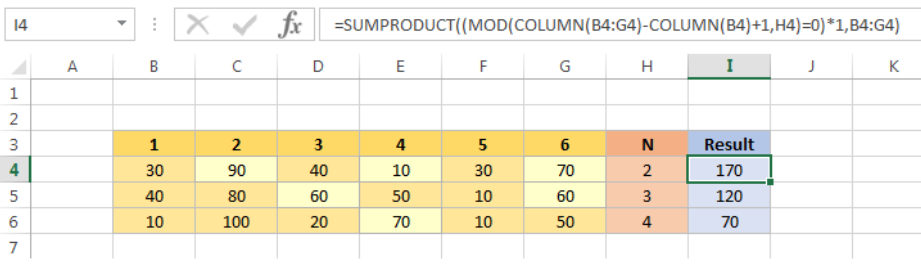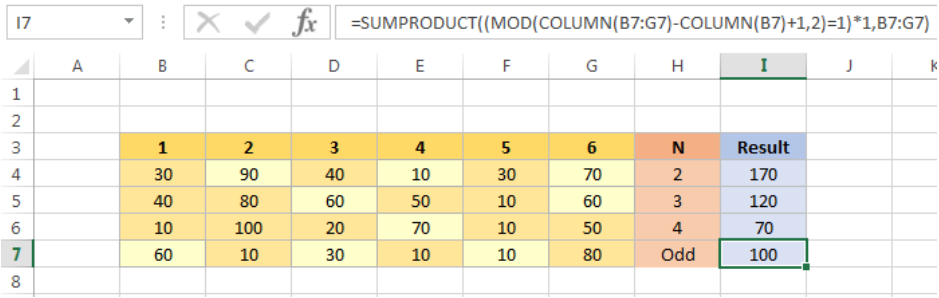Get instant live expert help with Excel or Google Sheets“My Excelchat expert helped me in less than 20 minutes, saving me what would have been 5 hours of work!”

#### Post your problem and you’ll get Expert help in seconds.

Your message must be at least 40 characters
Our professional Expert are available now. Your privacy is guaranteed.

# Sum every nth columnRead time: 20 minutes

You can use SUMPRODUCT, MOD and COLUMN functions to filter every nth column and sum the values in those columns.

# Sum every nth column

Formula

`= SUMPRODUCT((MOD(COLUMN(range)-COLUMN(range.first_column)+1,N)=0)*1,range)`

# Explanation

Range: Required. This range includes the values you want to sum.
Range.first_column: Required. This cell locates the first column of the range.
N: Required. This help to filter Nth column in the range.

In this formula, COLUMN functions get the relative column of the range. MOD function filters if the column number is divisible by N and returns an array of TRUE/FALSE elements. Finally, SUMPRODUCT will multiply and sum the equivalent values in the range that match TRUE returns in the array.

Example 1

Below is some of the example of using this formula. The highlighted values in the range B4:G4 help you to spot the Nth columns.Figure 1 – Sum every nth column

In I4, the formula is

`=SUMPRODUCT((MOD(COLUMN(B4:G4)-COLUMN(B4)+1,H4)=0)*1,B4:G4)`

First, COLUMN(B4:G4)-COLUMN(B4)+1,H4 portion is used to get relative column number is the range. It can be seen like this in arrays: {2,3,4,5,6,7} – 2 + 1 = {1,2,3,4,5,6}
Second, MOD(COLUMN(B4:G4)-COLUMN(B4)+1,H4)=0) returns and TRUE/FALSE array to filter the column number that divisible by H4 value. The array in this case is:{FALSE,TRUE, FLASE,TRUE, FLASE,TRUE} because {2,4,6} are divisible by H4=2. Multiplying this array by 1 (*1) will return an array like this: {0,1,0,1,0,1}.

Last, SUMPRODUCT uses the above array as the argument whose components you want to add and return the result.

## Notes

You can adapt this formula to sum even or odd columns like this:

`=SUMPRODUCT((MOD(COLUMN(B7:G7)-COLUMN(B7)+1,2)=1)*1,B7:G7)`Figure 2 – Sum every odd column

### Did this post not answer your question? Get a solution from connecting with the expert.Another blog reader asked this question today on Excelchat:
Solution exampleshow to make a format that if multiple cells have number contents its value is 1 in the last cell
Solved by C. C. in 28 minsI need to find out if the 2018 yearly sales goals were met if the yearly sales were \$25,000 or more using an IF logical function and to set the formula to return a value of YES if met, and NO if not
Solved by A. A. in 18 minsI'm looking for a formula or format for if there is data in c4 on a sheet... It adds extra information underneath
Solved by X. E. in 20 minsI am trying to put in an IF formula and although it works on all the other excel sheets I've worked on it is impossible to get it started in this new tab. The problem would be : =IF(G9="ENT.PSAB",N9,"") It simply doesn't do anything, when what I want is the debit amount to be repeated in another column with the same amount. Save typing in manually.
Solved by C. J. in 60 minsï?·In cell G1enter Discount, ï?·In cell H1enter DiscountPercent,ï?·In cell I1enter DiscountCost,ï?·In cell J1enter TotalCost, andï?·In cell K1enter Paymentas column headings.
Solved by K. E. in 40 mins## Subscribe to Excelchat.coAnother blog reader asked this question today on Excelchat: